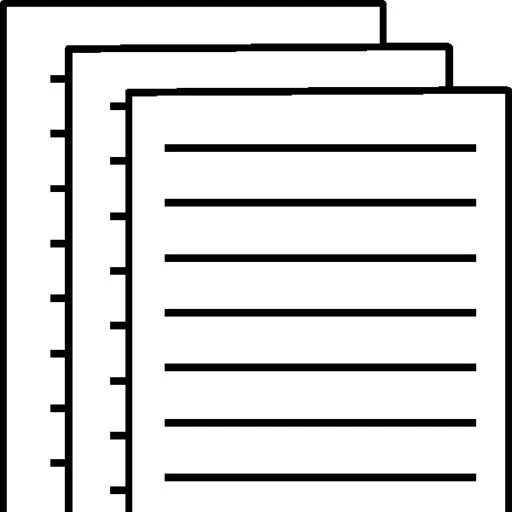## Inventory errors – Problem type 1

Inventory errors: Problem type 1

As shown in the chart above, errors in calculating the ending inventory cost have a direct effect on gross profit and net income. Errors in calculating the beginning inventory cost have an inverse effect on gross profit and net income.

Note that the effects of errors in calculating beginning and ending inventory costs have an opposite effect on the cost of goods sold. As shown in the chart above, errors in calculating the ending inventory cost have an inverse effect on the cost of goods sold. Errors in calculating the beginning inventory cost have a direct effect on the cost of goods sold.

Two examples of these types of errors are described below:

If the ending inventory cost is overstated

If the ending inventory cost is overstated, then the cost of goods sold, an expense, is understated. If an expense is understated, net income (and gross profit) will be overstated. Hence, the error in the ending inventory cost has a “carry-through” effect on net income and gross profit and an inverse effect on the cost of goods sold.

If the beginning inventory cost is overstated

If the beginning inventory cost is overstated, then the cost of goods sold, an expense, is overstated. If an expense is overstated, net income (and gross profit) will be understated. Hence, the error in the beginning inventory cost has an inverse effect on net income and gross profit and a “carry-through” effect on the cost of goods sold.

Cost of goods sold

Errors in beginning inventory have a direct effect on cost of goods sold, so cost of goods sold will be understated by \$4,700.

Errors in ending inventory have an inverse effect on cost of goods sold, so cost of goods sold will be overstated by \$6,400.

Taking both errors into consideration, cost of goods sold is overstated by \$1,700.

To view and download a complete answer, scroll down to the bottom to pay